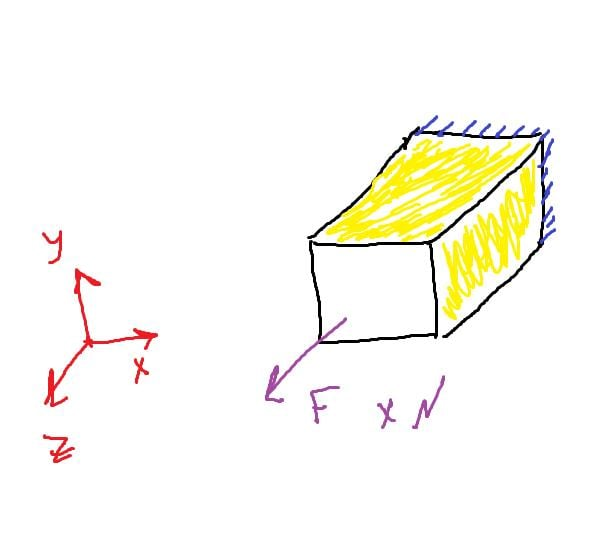## General Mechanical

Topics relate to Mechanical Enterprise, Motion, Additive Print and more

•bolbol
Subscriber

Hello,

My following question regarding the theory behind the displacement boundary condition.The following bar under:

- Applied force F in the z-direction on one of its ends.

- The other end is fixed.

- Applying displacement condition on the 4 yellow faces (2 of them is visible in the picture) with x=0,y=0, z=free.

And we know from Poisson's ratio that increasing the deformation in z-direction will lead to decreasing in the x and y-direction.

So, will my displacement condition which applied on the yellow faces violate the Poisson's ration?

•peteroznewman
Subscriber

Poisson's Ratio is the ratio of strain in the transverse direction divided by strain in the axial direction, when there is no constraint on lateral strain.  When that is the case, there is no stress in the transverse direction.

If you make a constraint preventing transverse strain, then a transverse tensile stress will develop.

Why are you asking here, why don't you just build the two models and look at the results?